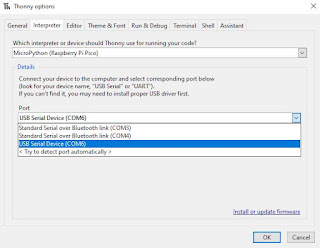## Shop## Tuesday, June 30, 2015

### HP 50g: Magnetism - Straight Wire & more

magnetic field is the magnetic effect of electric currents and magnetic materials

In this blog, using the HP 50g, I will show you how to get to the built in equation library and solve equations for
straight wire magnetism. The units for such is Tesla.
If you are required to answer in Gauss you will need to convert.
After turning on your HP 50g:
1. Press APPS
2. Scroll down to #12 or type in 12 (Equation Library)
3. Press OK. If Soft Menu mode is on you will see four boxes. Eqlib  Colib Mes Utils. Choose EQLIB. This is for the equation library.
4. In the library there are many choices. Today we will explore Magnetism. Go ahead and scroll down, press ENTER.
5. This brings us to today's topic. Straight Wire. Press ENTER. You should now see the see the equation. The soft key choices are now
• Solv Equ Vars Pic -->Stk Exit

1. PIC will show you a visual of the straight wire equation.
2. Vars gives a description of each variable that can be used in the equation.
3. EQN is the equation itself. The --> Stk puts the equation onto the stack.
4. The main soft key choice is Solv. This is the equation solver. To enter numerical information you must first key in the constant ex: 5_cm radius and 4_A current; -press 5 then press F2B the key directly under the r soft key menu, then 4 and F4Dfor current...soft key menu I.
5. Now to solve for B we must first press the White Right Shift key <-- and then F5E (B soft key menu)
Then solver should output the answer. Play around with different equations to get a feel for the powerful HP 50g!

Please subscribe for more great content.